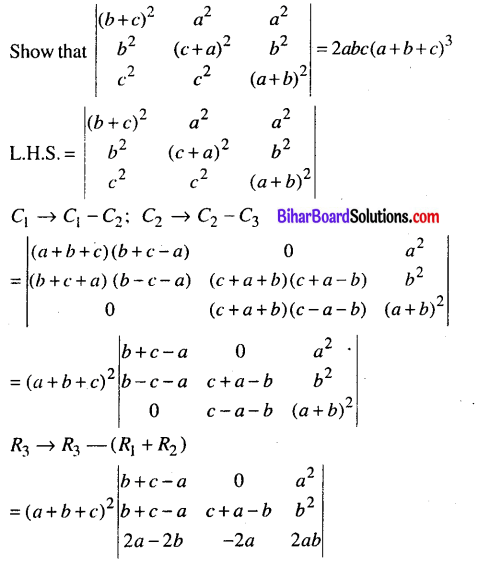# Bihar Board 12th Maths Model Question Paper 1 in English Medium

Bihar Board 12th Maths Model Papers

## Bihar Board 12th Maths Model Question Paper 1 in English Medium

Time : 3 Hours 15 Min
Full Marks: 100

Instructions for the candidates :

1. Candidates are required to give their answers in their own words as far as practicable.
2. Figure in the right-hand margin indicates full marks.
3. While answering the questions, the candidate should adhere to the word limit as far as practicable.
4. 15 Minutes of extra time has been allotted for the candidate to read the questions carefully.
5. This question paper is divided into two sections. Section-A and Section-B
6. In Section A, there are 1-50 objective type questions which are compulsory, each carrying 1 mark. Darken the circle with blue/black ball pen against the correct option on the OMR Sheet provided to you. Do not use Whitener/Liquid/ Blade/Nail on OMR Sheet otherwise result will be invalid.
7. In section-B, there are 25 short answer type questions (each carrying 2 marks), out of which only 15 (fifteen) questions are to be answered.
A part from this there is 08 Long Answer Type questions (each carrying 5 marks), out of which 4 questions are to be answered.
8. Use of any electronic device is prohibited.

Objective Type Questions

There are 1 to 50 objective type questions with 4 options, choose the correct option which, is to be answered on OMR Sheet. (50 x 1 = 50)

Question 1.
Which of the following has its inverse function one-one and onto?
(a) one-one onto
(b) one-one into
(c) many one onto
(d) many on into
(a) one-one ontoQuestion 2.
A relation R in a set X is an equivalence relation if R is
(a) reflexive
(b) symmetric
(c) transitive
(d) All of above
(d) All of above

Question 3.
The number of all one-one functions from set {a, b, c, d} to itself is
(a) 12
(b) 24
(c) 36
(d) None
(b) 24

Question 4.
If $$-\frac{\pi}{2}<x<\frac{\pi}{2}$$ then tan(tan-1 x) =
(a) tan x
(b) cotx
(c) x
(d) -x
(c) xQuestion 5.
Sin-1 x + Cos-1 y =
(a) 0
(b) $$\frac{\pi}{4}$$
(c) $$\frac{\pi}{2}$$
(d) π
(c) $$\frac{\pi}{2}$$

Question 6.
If x > 0, y > 0, xy < 1, then tan-1x + tan-1 y =
(a) tan-1 (x+y)
(b) $$\tan ^{-1} \frac{x+y}{1-x y}$$
(c) tan-1
(d) sin-1(x + y)
(b) $$\tan ^{-1} \frac{x+y}{1-x y}$$

Question 7.
(a) tan-12x
(b) $$\tan ^{-1} \frac{2 x}{1-x^{2}}$$
(c) $$\tan ^{-1} \frac{2 x}{1+x^{2}}$$
(d) $$\cot ^{-1} \frac{2}{x}$$
(b) $$\tan ^{-1} \frac{2 x}{1-x^{2}}$$

Question 8.
$$2\left[\begin{array}{ll} x & y \\ 1 & m \end{array}\right]$$ =(c) $$\left[\begin{array}{cc} 2 x & 2 y \\ 1 & 2 m \end{array}\right]$$Question 9.
$$5\left|\begin{array}{ll} 2 & 3 \\ 3 & 4 \end{array}\right|=$$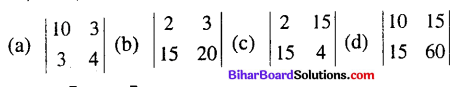$$\left|\begin{array}{cc} 2 & 3 \\ 15 & 20 \end{array}\right|$$

Question 10.
If A = $$\left[\begin{array}{cc} 5 & -5 \\ 5 & -5 \end{array}\right]$$ then A’ =(c) $$\left[\begin{array}{cc} 5 & 5 \\ -5 & -5 \end{array}\right]$$

Question 11.
$$\left|\begin{array}{ll} 1 & 0 \\ 0 & 1 \end{array}\right|=$$
(a) 0
(b) 1
(c) -1
(d) 2
(b) 1

Question 12.
$$\left[\begin{array}{ll} \mathbf{a} & \mathbf{b} \\ \mathbf{c} & \mathbf{d} \end{array}\right]+\left[\begin{array}{ll} \mathbf{p} & \mathbf{q} \\ \mathbf{r} & \mathbf{s} \end{array}\right]=$$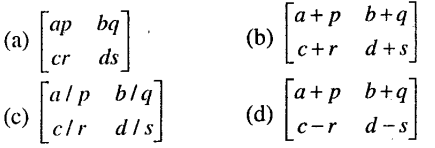$$\left[\begin{array}{cc} a+p & b+q \\ c+r & d+s \end{array}\right]$$

Question 13.
For a non-invertible matrix A,
(a) | A | = 0
(b)|A|≠ 0
(c) | A | = 1
(d) | A | = 2
(b)|A|≠ 0

Question 14.
If 1 is an unit matrix of order 2 × 2 then I3 =
(a) 3I2
(b) 3 + I
(c) 3I
(d) I
(d) IQuestion 15.
If A = $$\left[\begin{array}{ll} 2 & 3 \\ 4 & 5 \end{array}\right]$$ and $$\left[\begin{array}{ll} 4 & 6 \\ 8 & 10 \end{array}\right]$$ then 2A – B =
(a) 
(b) [0,0]
(c) $$\left[\begin{array}{ll} 0 & 0 \\ 0 & 0 \end{array}\right]$$
(d) 
(c) $$\left[\begin{array}{ll} 0 & 0 \\ 0 & 0 \end{array}\right]$$

Question 16.
If the order of two matrices A and B are 2×4 and 3×2 respectively then the order of AB is
(a) 2 x 2
(b) 4 x 3
(c) 2 x 3
(d) it is not possible to find AB
(d) it is not possible to find AB

Question 17.
$$\frac{d}{d x}(\tan x)=$$ =
(a) cot x
(b) Sec2x
(c) Secx tanx
(d) Secx
(b) Sec2x

Question 18.
$$\frac{d}{d x}\left(\sin ^{3} x\right)=$$
(a) 3 cos3
(b) 3 sin2xcosx
(c) 3sin2x
(d) cos3x
(b) 3 sin2xcosx

Question 19.
$$\frac{d}{d x}$$ [3 (sin2 x + cos2x )] =
(a) 3
(b) 1
(c) 0
(d) 6 sin x cos x
(c) 0

Question 20.
$$\frac{d}{d x}$$ (e4x) =
(a) e4x
(b) ex
(c) $$\frac{e^{4 x}}{4}$$
(d) 4e4x
(d) 4e4xQuestion 21.
$$\frac{d}{d x}\left(x^{5}\right)=$$
(a) 5x5
(b) 5x4
(c) $$\frac{x^{6}}{6}$$
(d) $$\frac{x^{4}}{4}$$
(b) 5x4

Question 22.
$$\frac{d}{d x}$$ [log(x3)] =
(a) $$\frac{1}{x^{3}}$$
(b) $$\frac{3}{x}$$
(c) 3x
(d) $$\frac{3}{x^{3}}$$
(b) $$\frac{3}{x}$$

Question 23.
If x= cosθ,y = sinθ then $$\frac{d y}{d x}$$ =
(a) tanθ
(b) sec2θ
(c) cotθ
(d) – cotθ
(d) – cotθ

Question 24.
$$\frac{d}{d x}\left(x^{1 / 3}\right)=$$(d) $$\frac{1}{3} x^{2 / 3}$$

Question 25.
∫ sin 2x dx =
(a) K + 2 cos2x
(b) $$\frac{\cos 2 x}{2}+K$$
(c) $$K-\frac{\cos 2 x}{2}$$
(d) $$K-\frac{\cos 2 x}{3}$$
(c) $$K-\frac{\cos 2 x}{2}$$

Question 26.
∫x4dx =
(a) K + x5
(b) $$K+\frac{x^{4}}{5}$$
(c) $$K+\frac{x^{5}}{5}$$
(d) $$K+\frac{x^{5}}{4}$$
(c) $$K+\frac{x^{5}}{5}$$

Question 27.
∫e3x dx =
(a) e3x + K
(b) K + 3e3x
(c) $$\frac{e^{3 x}}{3}+K$$
(d) $$\frac{e^{3 x}}{4}+K$$
(c) $$\frac{e^{3 x}}{3}+K$$Question 28.
$$\int \frac{3}{x} d x=$$
(a) K + 3x2
(b) $$K-\frac{3}{x^{2}}$$
(c) 3x + K
(d) K + 3 log|x|
(d) K + 3 log|x|

Question 29.
∫3dx =
(a) 3 + K
(b) x + K
(c) 3x + K
(d) 3K
(c) 3x + K

Question 30.
∫√x.dx =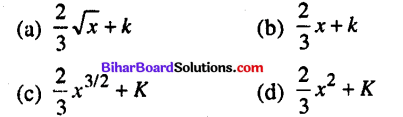(c) $$\frac{2}{3} x^{3 / 2}+K$$

Question 31.
$$\int_{0}^{\pi / 2} \sin x d x=$$
(a) 0
(b) 1
(c) -1
(d) $$\frac{\pi}{2}$$
(b) 1

Question 32.
$$\int_{0}^{1} e^{x} d x=$$
(a) e
(b) e + 1
(c) e – 1
(d) 2e
(c) e – 1

Question 33.
The solution of the equation $$\frac{d x}{x}=\frac{d y}{y}$$ is
(a) x = Ky
(b) xy = K
(c) x + y = K
(d) x – y = K
(a) x = Ky

Question 34.
The solution of the differential equation cosx dx + cosy dy = 0 is
(a) sinx + cosy = K
(b) sinx + siny = K
(c) cosx + cosy = K
(d) None of these
(b) sinx + siny = K

Question 35.
The solution of exdx + eydy = 0
(a) ex + ey= K
(b) ex – ey = K
(c) ex+y = K
(d) None of these
(a) ex + ey= K

Question 36.
The integrating factor of the linear differential equation $$\frac{d y}{d x}+x y=x^{3}$$ is
(a) ex
(b) $$e^{\frac{x^{2}}{2}}$$
(c) x
(d) None of these
Answre:
(b) $$e^{\frac{x^{2}}{2}}$$

Question 37.
∫ logdx =
(a) x log x – x + K
(b) x log x + x + K
(c) $$\frac{1}{x}+K$$
(d) $$\frac{1}{2}(\log x)^{2}+K$$
(a) x log x – x + KQuestion 38.
$$\int \frac{d x}{1+x^{2}}=$$
(a) tan-1 x + K
(b) sin-1 x + K
(c) cos-1 x + K
(d) cot-1 x + K
(a) tan-1 x + K

Question 39.
$$\overrightarrow{| \vec{i}} |=$$
(a) 0
(b) 1
(c) 2
(d) 3
(b) 1

Question 40.
$$\vec{i} \cdot \vec{i}=$$
(a) 0
(b) 1
(c) $$\vec{j}$$
(d) $$\vec{k}$$
(b) 1

Question 41.
$$\vec{i} \times \vec{i}=$$
(a) $$\vec{i}$$
(b) $$\vec{0}$$
(c) $$\vec{j}$$
(d) $$\vec{k}$$
(b) $$\vec{0}$$

Question 42.
If O is the origin and the position vector of a point(2,3,4) then $$\overrightarrow{\mathrm{OA}}=$$(b) $$2 \vec{i}+3 \vec{j}+3 \vec{k}$$

Question 43.
$$\overrightarrow{| i}+2 \vec{j}+3 \vec{K} |=$$
(a) 14
(b) 6
(c) 1
(d) √14
(d) √14

Question 44.
$$(2 \vec{i}-3 \vec{j}+4 \vec{k}) \cdot(3 \vec{i}+4 \vec{j}-5 \vec{k})=$$
(a) 14
(b) -14
(c) 26
(d) -26
(d) -26Question 45.
The direction cosines of the y-axis are
(a) 0, 0, 0
(b) 1, 0, 0
(c) 0, 1, 0
(d) 0, 0, 1
(c) 0, 1, 0

Question 46.
The condition for two lines having direction cosines I1 m1,n1 and I2, m2, n2 being parallel is
(a) l1l2 + m1m2 + n1n2 = 0
(b) $$\frac{I_{1}}{I_{2}}+\frac{m_{1}}{m_{2}}+\frac{n_{1}}{n_{2}}=0$$
(c) $$\frac{I_{1}}{I_{2}}=\frac{m_{1}}{m_{2}}=\frac{n_{1}}{n_{2}}$$
(d) None of these
(c) $$\frac{I_{1}}{I_{2}}=\frac{m_{1}}{m_{2}}=\frac{n_{1}}{n_{2}}$$

Question 47.
The equation of a plane parallel to the plane x + 2y + 3z + 5 = 0 is
(a) x + 2y + 3z + 5 = 0
(b) x – 2y + 3z + 5 = 0
(c) x + 2y – 3z + 5 = 0
(d) None of these
(d) None of these

Question 48.
The equation of a plane parallel to yz-plane is
(a) x + K
(b) y = K
(c) z = K
(d) None of these
(a) x + K

Question 49.
If A and B be two arbitrary events where A ≠ φ then P (A∩B) =
(a) P (A). P (B/A)
(b) P (A) + P (B/A)
(c) P (A) – P (B/A)
(d) None of these
(a) P (A). P (B/A)

Question 50.
The function in a linear programming problem whose maximum or minimum value has to be determined is called
(a) Objective function
(b) Constraint
(c) Both (a) and (b)
(d) None of these
(a) Objective function

Non-Objective Type Questions

Question No. 1 to 25 are short answer type questions. Each question of this category carries 2 marks. Answer any 15 questions. (15 x 2 = 30)

Question 1.
Examine whether the function f: R → R is one-one or many-one where f (x) = | x |, x ∈ R
We have f(-1) = |- 1| = 1 and f(-1) = | 1 | = 1
Thus two different elements in R have the same Image,
∴ f is not one-one function, f is many one function.

Question 2.
Prove that 2 $$\tan ^{-1} \frac{1}{5}+\tan ^{-1} \frac{1}{4}=\tan ^{-1} \frac{32}{43}$$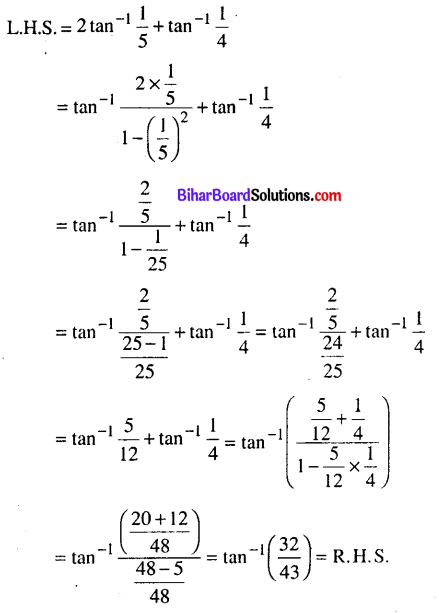Question 3.
Solve for x : cot-1 x + sin -1$$\frac{1}{\sqrt{5}}$$ = $$\frac{\pi}{4}$$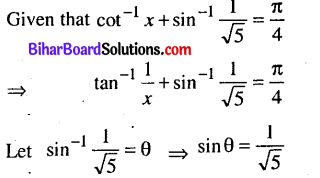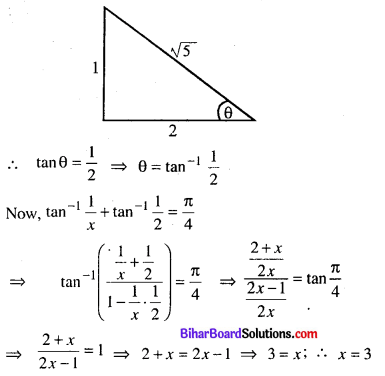Question 4.
Find the value of x from the following
$$\left[\begin{array}{cc} 2 x-y & 5 \\ 3 & y \end{array}\right]=\left[\begin{array}{cc} 6 & 5 \\ 3 & -2 \end{array}\right]$$
Given that $$\left[\begin{array}{cc} 2 x-y & 5 \\ 3 & y \end{array}\right]=\left[\begin{array}{cc} 6 & 5 \\ 3 & -2 \end{array}\right]$$
∴ 2x – y = 6 ………..(1)
y = -2………..(2)
from (i) 2x + 2 = 6 ⇒ 2x = 4. ∴ x = 2
∴x = 2, y = 2Question 5.
Evaluate:
$$\left|\begin{array}{ccc} 16 & 9 & 7 \\ 23 & 16 & 7 \\ 32 & 19 & 13 \end{array}\right|$$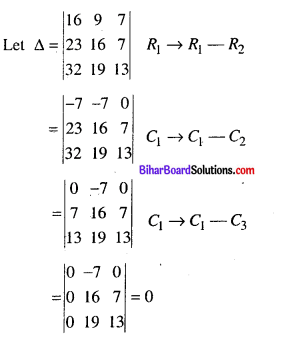Question 6.
When Evaluate x : $$\left|\begin{array}{ll} x & 4 \\ 2 & 2 x \end{array}\right|=0$$
Given that $$\left|\begin{array}{ll} x & 4 \\ 2 & 2 x \end{array}\right|=0$$
⇒ 2x2 – 8 = 0
⇒ 2x2 = 8
⇒ x2 = 4
∴ x = ±2

Question 7.
If A = $$\left[\begin{array}{c} 2 \\ -4 \\ 3 \end{array}\right]$$ and B = [ 2 3 4] then find B’A’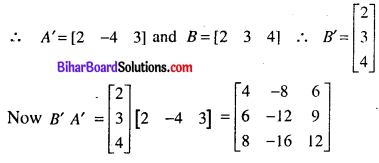Question 8.
If y + x = sin (y +x) then find dy/dxQuestion 9.
If $$y=\log \left(x^{2} \sqrt{x^{2}+1}\right)$$ then find $$\frac{d y}{d x}$$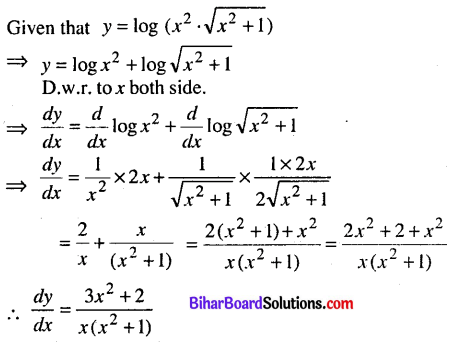Question 10.
If x = a cosθ, y = b sinθ, then find dy/dx
Given that x = acosθ
D.W.R. to θ $$\frac{d x}{d \theta}$$ = -asinθ…(i)
and y = bsinθ
D.w.r. to θ ; $$\frac{d y}{d \theta}$$ = bcosθ …(ii)
$$\frac{(\mathrm{ii})}{(\mathrm{i})} \frac{d y}{d x}=-\frac{b}{a} \cot \theta$$

Question 11.
Integrate ∫ (sin x +cos x)2dx.
Let I = ∫ (sin x + cos x)2 dx
= ∫ (sin2x + cos2x + 2sinx-cosx)dlv
= ∫ (l + sin 2x)clx = ∫ dx + ∫ sin 2x dx
= $$x-\frac{\cos 2 x}{2}+c$$

Question 12.
Evaluate: $$\int_{0}^{\pi / 2} \frac{d x}{1+\sin x}$$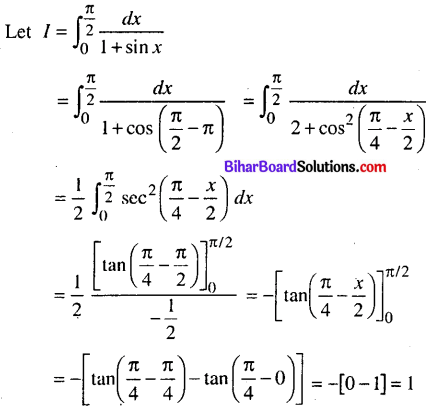Question 13.
Evaluate : $$\int_{0}^{\pi / 2} \frac{\sin x d x}{\sin x+\cos x}$$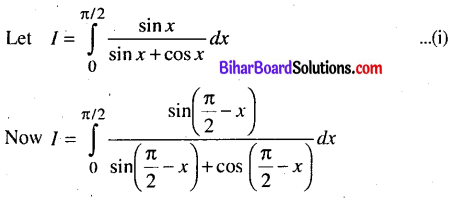Question 14.
Solve $$\frac{d y}{d x}$$ – y tanx =-ysec2x.
Given differentia] Equation is $$\frac{d y}{d x}$$ – y tanx =-ysec2x.
This is L.D.E. of the form $$\frac{d y}{d x}$$ + Py = Q
Here p = – tan x Q = sec2x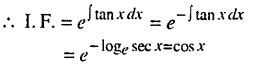∴ Solution of given diff. eqn. is
y x l.F.= ∫ Q-(lF)dx + C
⇒ ycosx = ∫sec2x.cosxdx + C
⇒ ycosx = ∫ secxdx + C
⇒ ycosx = log |secx + tanx| + C
This is requried solution of given diff. eqn.

Question 15.
Integrate : ∫x2exdx
Let I = ∫x2exdx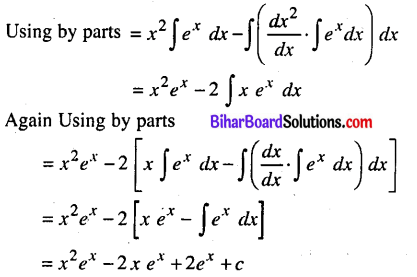Question 16.
Find the scalar product of $$\overrightarrow{5} \vec{i}+\vec{j}-3 \vec{k}$$ and $$3 \vec{i}-\overrightarrow{4 j}+7 \vec{K}$$
Let $$\vec{a}=5 \hat{l}+\hat{j}-3 \hat{k}$$ and $$\vec{b}=3 \hat{\imath}-4 \hat{j}-7 \hat{k}$$
∴ $$\vec{a} \cdot \vec{b}$$ = (5 x 3) + (1 x -4)÷(- 3 x 7)
15 – 4 – 21 = – 10

Question 17.
If $$\vec{a}=3 \vec{i}+4 \vec{j}-5 \vec{k}$$ and b = $$7 \vec{i}-3 \vec{j}+6 \vec{K}$$ then find $$(\vec{a}+\vec{b}) \times(\vec{a}-\vec{b})$$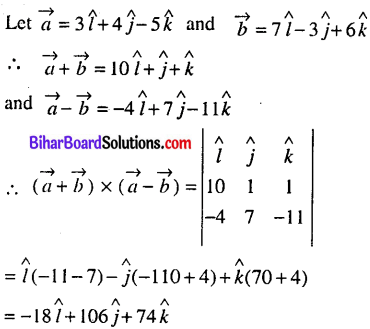Question 18.
Find the acute angle between two straight lines whose direction ratios are (1,1,0) and (2,1,2).
Direction ratios of the first line are 1,1,0
∴Its direction cosines are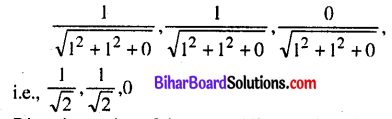Direction ratios of the second line are 2,1,2
its direction cosines are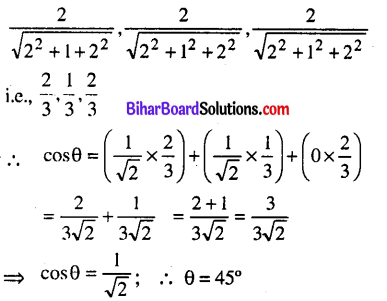Question 19.
Find the values of p so that the lines $$\frac{11-x}{p}=\frac{3 y-3}{2}=\frac{17-z}{5}$$ and
$$\frac{x-22}{3 p}=\frac{2 y-7}{27 p}=\frac{z-100}{6 / 5}$$ are perpendicular to each other.⇒ -3p2 + 9p – 6 = 0
⇒ 3p2 – 9p + 6 = 0
⇒ p2 – 3p + 2 = 0
⇒ p2 – 2p – p + 2 = 0
⇒ p(p – 2) -1 (p – 2) =0
⇒ (p – 2) (p – 1) = 0
∴ p = 2, 1

Question 20.
Prove that the two planes, 3x – 4y + 5z = 0 and 2x – y – 2z = 5 are mutually perpendicular.
Given equations of plane are 3x – 4y + 5z = 0 …(i)
and 2x-y-2z = 5 …(ii)
Here a1= 3 b1= – 4, c1 = 5; a2 = 2, b2 = -1 c2=- 2
a1a2 + b1b2 + c1c2; 6 + 4 – 10 = 0
Since product of Direction’ratios at two planes are zero, plane (i) & (ii) are perpendicular.Question 21.
What is the probability of occurrence of a number greater than 2 if it is known that only even num¬bers can occur ?
Let S = {1,2,3,4,5,6}; A = {2,4,6}
B= {3, 4, 5,6}Question 22.
A person tosses a coin 3 times. Find the probability of occurrence of exactly one head.
Let p = Probability of getting a head in one trialQuestion 23.
If y = sin x + cosx, then find $$\frac{d^{2} y}{d x^{2}}$$
Answre:
Given that y = sinx + cosx; D.w.r. to x both sides
∴ $$\frac{d y}{d x}$$ = cps x – sin x, Again D.w.r.to x both sides
⇒ $$\frac{d^{2} y}{d x^{2}}$$ = – sin x – cosx (sinx + cosx)

Question 24.
Find the values of $$\left|\begin{array}{lll} a & a^{2} & a^{3} \\ b & b^{2} & b^{3} \\ c & c^{2} & c^{3} \end{array}\right|$$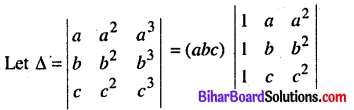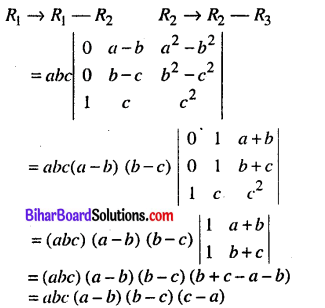Question 25.
If A and B be two events and 2P (A) = P (B) = 6/13 and P (A/B) = 1/3, then find (P(A ∪ B)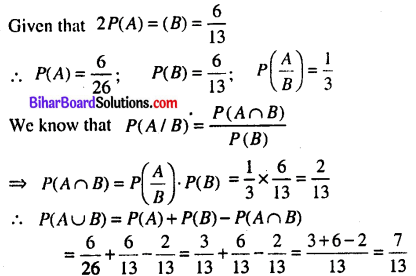Question no. 26 to 33 are long answer type questions. Each question carries 5 marks.
Answer any 4 questions out of these. (4 × 5 = 20)

Question 26.
If y = $$e^{x^{x}}$$ then find $$\frac{d y}{d x}$$Question 27.
Prove that sinθ (1 + cosθ)has maximum value at θ = $$\frac{\pi}{3}$$
Let y = sinθ(1 + cosθ)
D .w.r. to 0 both sidesQuestion 28.
Evaluate : $$\int_{0}^{\pi} \frac{x}{1+\sin x} d x$$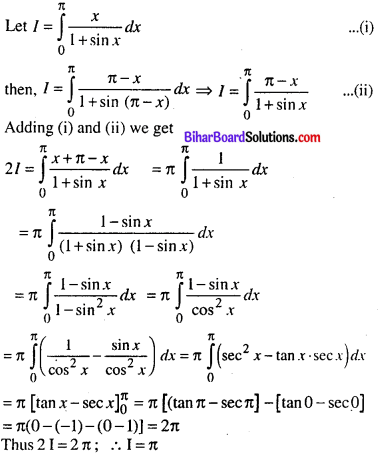Question 29.
Solve: (x2 + y2)$$\frac{d y}{d x}$$ = 2xy
Given differential equ. is (x2 + y2)$$\frac{d y}{d x}$$ = 2xyThis is Homogeneons diff. equation put y = vx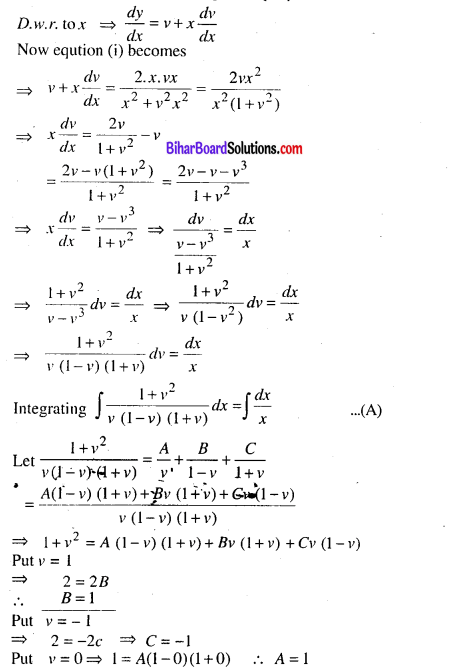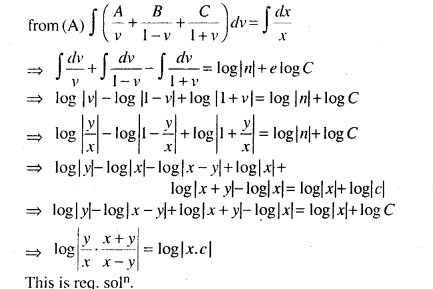Question 30.
Maximize : Z = 50x + 15y
subject to : 5A + y ≤ 5, x + y ≤ 3 and x, y ≥ 0
Its corresponding equation
5x + y = 5 …… (i)
x + y = 3 ……..(ii)Question 31.
A speaks the truth in 75% casses and B in 80% of the cases. In what percentage of cases are they likely to contradict each other in stating the same fact ?
Let E = The Event of A speaking the truth and F = The Event of B speaking the truth.
Then P(E) = $$\frac{75}{100}=\frac{3}{4}$$ and P(F) = $$\frac{80}{100}=\frac{4}{5}$$
Required probability P(A & B contradicting each other)
= P(EF̄) or ĒF) = P(EF̄+ĒF)= P(E)- P(F̄) + P(Ē).P(F)
= P(E) .[1 – P(F)]+[1 – P(E). P(F)]
∴ A &.B are likely to contradict each other in 35% cases.

Question 32.
Find the acute angle between the straight line $$\frac{x}{1}=\frac{y}{3}=\frac{z}{0}$$ and plane 2x + y = 5
Given that $$\frac{x}{1}=\frac{y}{3}=\frac{z}{0}$$ and 2x + y = 5
Here a1, b1, c1 & a2 = 2, b2= 1, c2 = 0
∴ Acute angle between the given line and plane is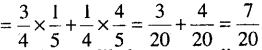Question 33.
Factorize. $$\left|\begin{array}{ccc} (b+c)^{2} & a^{2} & a^{2} \\ b^{2} & (c+a)^{2} & b^{2} \\ c^{2} & c^{2} & (a+b)^{2} \end{array}\right|$$